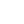# Advent of Code 2022 - Day 2 Javascript Solution

By Henri ParviainenAdvent of Code day 2 is here with a new puzzle. Let's find out how to solve it with Javascript.

## Disclaimer

This solution is not the fastest implementation nor is it claiming to be the best way to solve the problem. There are many ways to solve it and this is just how I did it with Javascript. By sharing I hope it can help someone who is struggling to find a solution to the puzzle.

## Solution

To be able to run my solution, you should have same project setup as me.

First, download the input and save it as a `txt` file in the same directory as you have your javascript files. If you don't yet have any javascript files - make two - one for each part.

So at the starting point, your folder structure should be:

``````/
-- part1.js
-- part2.js
-- input.txt``````

### Part 1

Add the following code to your `part1.js` file.

``````let fs = require("fs");

const playsArray = input.split("\n");

let myPoints = 0;

for (let play of playsArray) {
let opponentPick = play.split(" ");
let myPick = play.split(" ");

// Opppoent picks rock

if (opponentPick == "A" && myPick == "Z") {
console.log("opponent picks rock and you pick scissors, you lost.");
myPoints = myPoints + 3 + 0;
}
if (opponentPick == "A" && myPick == "X") {
console.log("opponent picks rock and you pick rock, it's a draw.");
myPoints = myPoints + 1 + 3;
}
if (opponentPick == "A" && myPick == "Y") {
console.log("opponent picks rock and you pick paper, you win.");
myPoints = myPoints + 2 + 6;
}

// Opponent picks paper

if (opponentPick == "B" && myPick == "Z") {
console.log("opponent picks paper and you pick scissors, you win.");
myPoints = myPoints + 3 + 6;
}
if (opponentPick == "B" && myPick == "X") {
console.log("opponent picks paper and you pick rock, you lose.");
myPoints = myPoints + 1 + 0;
}
if (opponentPick == "B" && myPick == "Y") {
console.log("opponent picks paper and you pick paper, its a draw.");
myPoints = myPoints + 2 + 3;
}

// Opponent picks scissors

if (opponentPick == "C" && myPick == "Z") {
console.log("opponent picks scissors and you pick scissors, its a draw.");
myPoints = myPoints + 3 + 3;
}
if (opponentPick == "C" && myPick == "X") {
console.log("opponent picks scissors and you pick rock, you win.");
myPoints = myPoints + 1 + 6;
}
if (opponentPick == "C" && myPick == "Y") {
console.log("opponent picks scissors and you pick paper, you lose.");
myPoints = myPoints + 2 + 0;
}
}

console.log(myPoints);``````

Run in it with `node part1.js` and you'll have your answer for the first part!

### Part 2

For the second part, add the following code to `part2.js`.

``````let fs = require("fs");

const playsArray = input.split("\n");

let myPoints = 0;

for (let play of playsArray) {
let opponentPick = play.split(" ");
let myPlan = play.split(" ");

// Plan is to lose

if (myPlan == "X") {
console.log("Plan is to lose.");

if (opponentPick == "A") {
console.log(
"opponent picks rock and plan is to lose. So, you pick scissors."
);
myPoints = myPoints + 3 + 0;
}
if (opponentPick == "B") {
console.log(
"opponent picks paper and plan is to lose. So, you pick rock."
);
myPoints = myPoints + 1 + 0;
}
if (opponentPick == "C") {
console.log(
"opponent picks scissors and plan is to lose. So, you pick paper."
);
myPoints = myPoints + 2 + 0;
}
}

// Plan is to draw

if (myPlan == "Y") {
console.log("Plan is to draw.");

if (opponentPick == "A") {
console.log(
"opponent picks rock and plan is to draw. So, you pick rock."
);
myPoints = myPoints + 1 + 3;
}
if (opponentPick == "B") {
console.log(
"opponent picks paper and plan is to draw. So, you pick paper."
);
myPoints = myPoints + 2 + 3;
}
if (opponentPick == "C") {
console.log(
"opponent picks scissors and plan is to draw. So, you pick scissors."
);
myPoints = myPoints + 3 + 3;
}
}

// Plan is to win

if (myPlan == "Z") {
console.log("Plan is to draw.");

if (opponentPick == "A") {
console.log(
"opponent picks rock and plan is to win. So, you pick paper."
);
myPoints = myPoints + 2 + 6;
}
if (opponentPick == "B") {
console.log(
"opponent picks paper and plan is to win. So, you pick scissors."
);
myPoints = myPoints + 3 + 6;
}
if (opponentPick == "C") {
console.log(
"opponent picks scissors and plan is to win. So, you pick rock."
);
myPoints = myPoints + 1 + 6;
}
}
}

console.log(myPoints);``````

And by running `node part2.js` you have the answer for the second part.